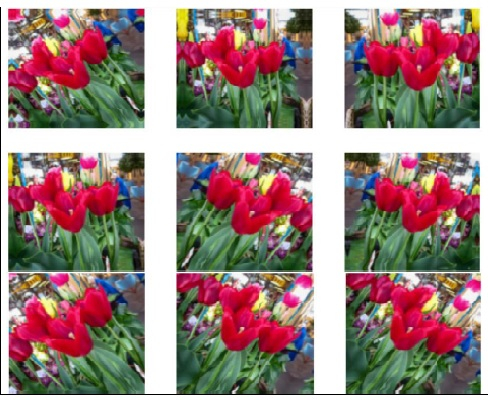# How can Tensorflow be used to visualize the augmented data from the dataset?

TensorflowServer Side ProgrammingProgramming

The augmented data can be visualized using Tensorflow and Python with the help of ‘matplotlib’ library. The images are iterated over, and plotted using ‘imshow’ method.

We will use the Keras Sequential API, which is helpful in building a sequential model that is used to work with a plain stack of layers, where every layer has exactly one input tensor and one output tensor.

A neural network that contains at least one layer is known as a convolutional layer. We can use the Convolutional Neural Network to build learning model.

We are using the Google Colaboratory to run the below code. Google Colab or Colaboratory helps run Python code over the browser and requires zero configuration and free access to GPUs (Graphical Processing Units). Colaboratory has been built on top of Jupyter Notebook.

When the number of training examples is small, the model learns from noises or unwanted details from training examples. This negatively impacts the performance of the model on new examples.

Due to overfitting, the model will not be able to generalize well on the new dataset. There are many ways in which overfitting can be avoided. We will use data augmentation to overcome overfitting.

Data augmentation generates additional training data from the existing examples by augmenting them with the help of random transformations that would yield believable-looking images.

This helps expose the model to more aspects of the data, thereby helping the model generalize better. Following is an example:

## Example

print("Visualizing the augmented examples")
plt.figure(figsize=(10, 10))
for images, _ in train_ds.take(1):
for i in range(9):
augmented_images = data_augmentation(images)
ax = plt.subplot(3, 3, i + 1)
plt.imshow(augmented_images.numpy().astype("uint8"))
plt.axis("off")

## Output

Visualizing the augmented examples## Explanation

• The above code helps visualize some augmented examples.

• This is done by applying data augmentation on the same image multiple times.

• The data/images is iterated over, and some of the sample images are displayed on the console.